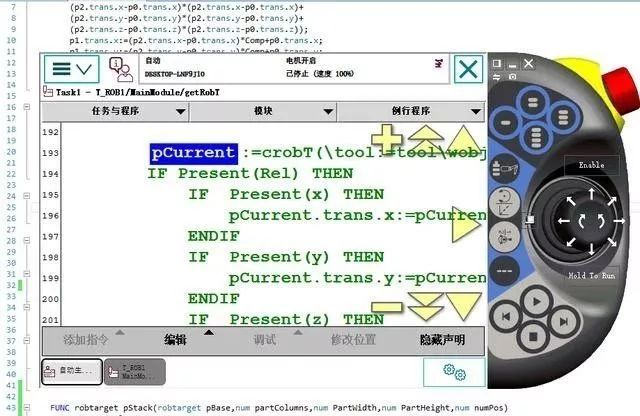# ABB機器人指令開發示例

### 0人氣：5

• 關鍵詞： ABB機器人指令
• 摘要：在編程的靈活性方面相比，ABB機器人比一般國產和日系機器人要方便很多，本文簡單介紹ABB機器人指令開發過程，其實也就是編寫附帶了參數的程序。

RAPID應用被稱作一項任務。一項任務包括一組模塊。一個模塊包含一組數據和程序聲明。

Atomic(內置原子型/基本數據類型)數據類型：

bool為枚舉類型，其值為真或假，提供了一種開展邏輯計算和關聯計算的方式。

num型支持精確算術計算和近似算術計算。

string型表示字符序列。

Record（記錄型）數據類型：為一種帶命名有序分量的復合類型。record類型的值為由各分量的值組成的復合值。一個分量可具備atomic型或record型，用戶自定義復雜數據類型一般就是記錄型。內置記錄型有：

pos型表示空間位置（矢量）。

orient型表示在空間中的方位。

pose型表示坐標系（位置/方位組合）。

alias數據類型被定義為等同于另一種類型。alias類型提供一種對象分類手段。系統可采用alias分類來查找和顯示與類型相關的對象，內置別名型數據有errnum和intnum。Errnum和intnum均為num的別名，用于表示錯誤和中斷編號。

Rapid程序分為三類：無返回值程序（PROC）、有返回值程序（FUNC）和軟中斷程序。程序聲明指定了程序的下列內容：名稱，數據類型（僅對有返回值程序有效），參數（不適用于軟中斷程序），數據聲明和語句（體），回退處理器（僅對無返回值程序有效），錯誤處理器和撤銷處理器

FUNC robtarget pStack(robtarget pBase,num partColumns,num partWidth,num PartHeight,num numCounter)

VAR num Columns;

VAR num Rows;

Columns:=( numCounter -1) MOD partColumns;

Rows:=( numCounter -1-Columns)/partColumns;

RETURN Offs(pBase,0,Columns*(PartWidth),Rows*PartHeight);

ENDFUNC

FUNC robtarget getRobT(switch Rel,num x,num y,num z,inout tooldata tool,wobjdata wobj)

VAR robtarget pCurrent;

pCurrent:=crobT(tool:=toolwobj:=wobj);

IF Present(Rel) THEN

IF Present(x) THEN

pCurrent.trans.x:=pCurrent.trans.x+x;

ENDIF

IF Present(y) THEN

pCurrent.trans.y:=pCurrent.trans.y+y;

ENDIF

IF Present(z) THEN

pCurrent.trans.z:=pCurrent.trans.z+z;

ENDIF

ELSE

IF Present(x) THEN

pCurrent.trans.x:=x;

ENDIF

IF Present(y) THEN

pCurrent.trans.y:=y;

ENDIF

IF Present(z) THEN

pCurrent.trans.z:=z;

ENDIF

ENDIF

RETURN pCurrent;

tENDFUNC

movel getRobPos(rel,y:=100, ,tool0,wobj0),v100,z0,tool0;

movel getRobPos(z:=1000,tool0,wobj0),v100,z0,tool0;

p1:= getRobPos(y:=200,tool0,wobj0),

FUNC bool rCheckPos(jointtarget pCheck,num num1)

VAR jointtarget pCurrent;

VAR num numTemp;

IF Present(num1) THEN

numTemp:=num1;

ENDIF

pCurrent:=CalcJointT(CRobT(),tool0);

IF pCurrent.robax.rax_1<=pcheck.robax.rax_1+num1 and="" pcurrent.robax.rax_1="">=pCheck.robax.rax_1-num1 AND

pCurrent.robax.rax_2<=pcheck.robax.rax_2+num1 and="" pcurrent.robax.rax_2="">=pCheck.robax.rax_2-num1 AND

pCurrent.robax.rax_3<=pcheck.robax.rax_3+num1 and="" pcurrent.robax.rax_3="">=pCheck.robax.rax_3-num1 AND

pCurrent.robax.rax_4<=pcheck.robax.rax_4+num1 and="" pcurrent.robax.rax_4="">=pCheck.robax.rax_4-num1 AND

pCurrent.robax.rax_5<=pcheck.robax.rax_5+num1 and="" pcurrent.robax.rax_5="">=pCheck.robax.rax_5-num1 AND

pCurrent.robax.rax_6<=pcheck.robax.rax_6+num1 and="" pcurrent.robax.rax_6="">=pCheck.robax.rax_6-num1 THEN

RETURN TRUE;

ELSE

RETURN FALSE;

ENDIF

ENDFUNC****** *** “三〇智工”——智能制造專注技術人才職業發展高端服務平臺。優質平臺更有實力保障，精準企業需求與人才技能匹配，更涵蓋最新的實習新趨勢、當地的產業經濟發展和人才政策推行帶來的實習紅利、當地的重點產業、優質企業實習人才需求缺口、企業選育留實習人才的偏好等信息資訊，無論你實習是追求學習機會，或是追求技能提升，或是職業發展，都可以滿足你的需求。

【更多信息請關注：三〇智工（微信號sanlingzhigong）】評價： 一般